# PSAT Math : How to find the length of the side of an acute / obtuse triangle

## Example Questions

2 Next →

### Example Question #561 : Geometry

All of the following could be the possible side lengths of a triangle EXCEPT: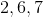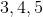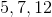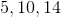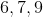Explanation:

The length of the third side of a triangle must always be between (but not equal to) the sum and the difference of the other two sides.

For instance, take the example of 2, 6, and 7.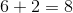and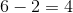. Therefore, the third side length must be greater than 4 and less than 8. Because 7 is greater than 4 and less than 8, it is possible for these to be the side lengths of a triangle.

The 5, 7, 12 answer choice is the only option for which this is not the case.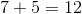and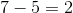. Therefore, the third side length must be between 2 and 12. Because it is equal to the sum, not less than the sum, it is not possible that these could be the side lengths of a triangle.

2 Next →

### All PSAT Math Resources